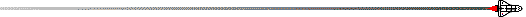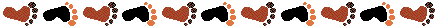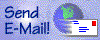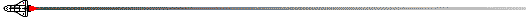# BEGINNING READINESS FOR SIXTH GRADE### The student should have a good grasp of multiplication facts 0-10 The student should be able to multiply and divide single digit numbers The student should have a solid understanding and working knowledge of addition and subtraction principles of borrowing and carrying The student should be able to add, subtract, multiply and divide fractions including finding the common denominator, lowest terms and simplest form The student should be able to do 60 multiplication facts in 60 seconds The student should demonstrate an understanding of place value for whole numbers to millions and decimals to thousandths The student should understand the process of addition, subtraction, multiplication and division of whole numbers and decimals with double and triple digits and regrouping The student should demonstrate an understanding of basic metric and stand measurement The student should understand simple geometry concepts including: angles, segments, vertices, shapes, area, perimeter, volume, radius, and diameter ## Language Readiness The student should know the proper use of verbs, nouns and adjectives in a sentence The student should be able to write in legible cursive The student should be able to distinguish between nouns, pronouns, verbs, adjectives, adverbs, and prepositions The student should be able to write complete sentences with proper capitalization and simple punctuation The student should be able to distinguish between simple and compound sentences, phrases and run-on sentences The student should be able to write a paragraph with topic sentence, at least three detail sentences and a summary sentence ## Reading Readiness The student should be able to follow simple instructions with understanding The student should be able to read independently both orally and silently The student should be able to analyze with understanding what they have read The student should be able to use an index, table of contents and glossary The student should be able to use a dictionary and an encyclopedia ## Social Studies and Science Readiness The student should be able to use simple information from maps, charts, tables, and diagrams The student should be able to distinguish between cities, states, countries, and continents The student should be able to understand hemispheres, parallels and meridians The student should be able to use textbooks to see whole ideas and details # "Repetition is the Key to memory"The most important question in life